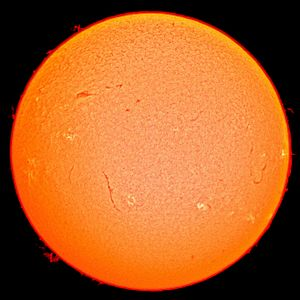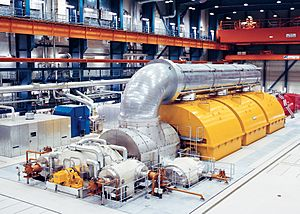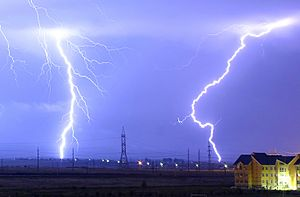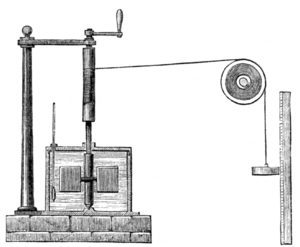# Energy facts for kids

Kids Encyclopedia FactsThe Sun is the source of energy for most of life on Earth. It derives its energy mainly from nuclear fusion in its core and releases it into space mainly in the form of radiant (light) energy.

Energy is a word with more than one meaning.

• Mostly it is used in science to describe how much potential a physical system has to change. In physics, energy is a property of matter. It can be transferred between objects, and converted in form. It cannot be created or destroyed.
• It may also be used in economics to describe the part of the market where energy itself is harnessed and sold to consumers.
• In ordinary language, the word is used to describe someone acting or speaking in a lively and vigorous way.

## Scientific energyA turbo generator transforms the energy of pressurised steam into electrical energy

In science energy is something that can do work.

There are two basic forms of energy:

### Conservation of Energy

Energy cannot be made or destroyed, it is changed from one form to another. The amount of energy in a closed system is always the same. This rule is called the "conservation law of energy".

### Conservation of matter and energy

Scientists now know that matter can be made into energy, and energy into matter, through processes like nuclear fission and nuclear fusion. The law of conservation of energy has therefore been extended to become the Law of conservation of matter and energy. Albert Einstein was the first to mathematically derive this in the formula E = mc2.

#### Example

A stone is thrown upwards and falls to the ground.

1. human throws the stone: energy store in muscles = chemical energy
2. stone moves upwards = kinetic energy
3. stone at the highest point = potential energy
4. stone falls to ground = kinetic energy
5. stone hits ground = thermal energy/sonic energy

### Types of energyIn a typical lightning strike, 500 megajoules of electric potential energy is converted into the same amount of energy in other forms, mostly light energy, sound energy and thermal energy.

The total energy of a system can be subdivided and classified in various ways. For example, classical mechanics distinguishes between kinetic energy, which is determined by an object's movement through space, and potential energy, which is a function of the position of an object within a field. It may also be convenient to distinguish gravitational energy, thermal energy, several types of nuclear energy (which utilize potentials from the nuclear force and the weak force), electric energy (from the electric field), and magnetic energy (from the magnetic field), among others. Many of these classifications overlap; for instance, thermal energy usually consists partly of kinetic and partly of potential energy.

Some types of energy are a varying mix of both potential and kinetic energy. An example is mechanical energy which is the sum of (usually macroscopic) kinetic and potential energy in a system. Elastic energy in materials is also dependent upon electrical potential energy (among atoms and molecules), as is chemical energy, which is stored and released from a reservoir of electrical potential energy between electrons, and the molecules or atomic nuclei that attract them.The list is also not necessarily complete. Whenever physical scientists discover that a certain phenomenon appears to violate the law of energy conservation, new forms are typically added that account for the discrepancy.

Heat and work are special cases in that they are not properties of systems, but are instead properties of processes that transfer energy. In general we cannot measure how much heat or work are present in an object, but rather only how much energy is transferred among objects in certain ways during the occurrence of a given process. Heat and work are measured as positive or negative depending on which side of the transfer we view them from.

Potential energies are often measured as positive or negative depending on whether they are greater or less than the energy of a specified base state or configuration such as two interacting bodies being infinitely far apart. Wave energies (such as radiant or sound energy), kinetic energy, and rest energy are each greater than or equal to zero because they are measured in comparison to a base state of zero energy: "no wave", "no motion", and "no inertia", respectively.

Examples:

### Measuring energyJoule's apparatus for measuring the mechanical equivalent of heat. A descending weight attached to a string causes a paddle immersed in water to rotate.

Energy can be measured. The amount of energy a thing has can be given a number.

As in other kinds of measurements, there are measurement units. The units of measurement for measuring energy are used to make the numbers meaningful.

#### Some scientific units of measurement

The SI unit for both energy and work is the joule (J). It is named after James Prescott Joule. 1 joule is equal to 1 newton-metre. In terms of SI base units, 1 J is equal to 1 kg m2 s−2.

The energy unit of measurement for electricity is the kilowatt-hour (kW·h). One kW·h is equivalent to 3,600,000 J (3600 kJ or 3.6 MJ)

## Related pagesEnergy Facts for Kids. Kiddle Encyclopedia.